# Electronics and Communication Engineering - Materials and Components

Exercise : Materials and Components - Section 6
1.
When an electron moves at velocity v in combined electric and magnetic fields E and H, the Lorentz force F is F = - e(E + v x B).
True
False
Explanation:
No answer description is available. Let's discuss.

2.
A parallel plate capacitor has area of plate equal to 1 m and distance between plates is also 1 m2. The lossy dielectric has a complex dielectric constant ∈r = ∈'r - j"r. If the capacitor is represented by R and C is parallel, then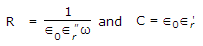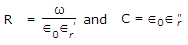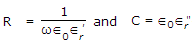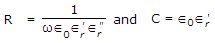Explanation:
No answer description is available. Let's discuss.

3.
When Schrodinger equation is applied to the motion of electron in the field of protons, the physically acceptable solutions for the wave function exist for quantum number which are
2
3
4
5
Explanation:

There are three principal quantum numbers.

4.
For dielectrics in alternating field, polarizability ae is a complex quantity. The imaginary part of ae is zero for
ω = 0
ω → ∞
ω = 0 and ω → ∞
ω = natural frequency ω0
Explanation:

Imaginary part of ae is zero for ω = 0 and ω = ∞.

5.
In the wave mechanical theory, the maximum of the charge distribution in the ground state occurs for a distance from the nucleus equal to
The total charge associated with an electron moving about nucleus in the ground state of hydrogen atom is given by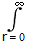4p2rr(r)dr.